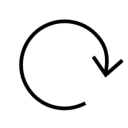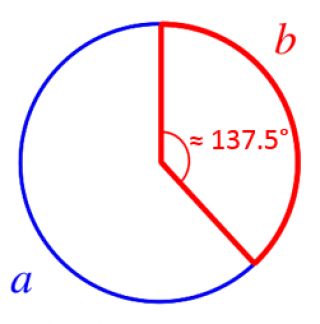◎◎◎ complex number ⪢⪢
A complex number is a number that can be expressed in the form a + bi where a and b are real numbers and i is the square root of -1. #math
http://qindex.info/i.php?x=9607
root ⪢⪢
The origins of what Putin wants today are rooted in what happened more than 3 decades ago back in the early 1990s when the Soviet Union first collapsed.
http://qindex.info/i.php?x=20196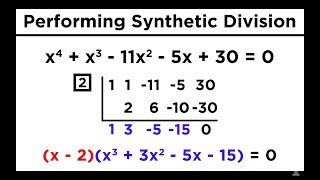16 Solving Higher Degree Polynomials by Synthetic Division and the Rational Roots Test
Solving Higher Degree Polynomials by Synthetic Division and the Rational Roots Test
http://qindex.info/i.php?x=5084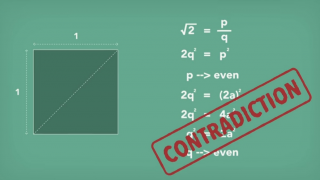BC 0500? Hippasus
According to Pythagoras theorem the diagonal length of a square with each side measuring one unit would be square root of two. The assumption that square root of two could be expressed as a ratio of two integers deduces a contradiction.
http://qindex.info/i.php?x=5026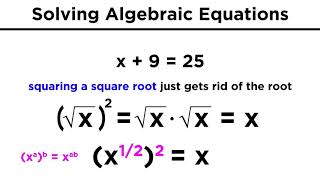09 Solving Algebraic Equations With Roots and Exponents
Solving Algebraic Equations With Roots and Exponents
http://qindex.info/i.php?x=380209 Solving Algebraic Equations With Roots and Exponents
Solving Algebraic Equations With Roots and Exponents
http://qindex.info/i.php?x=4994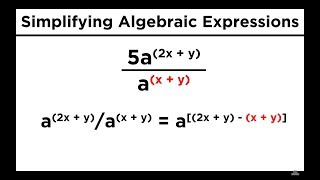08 Simplifying Expressions With Roots and Exponents
Simplifying Expressions With Roots and Exponents
http://qindex.info/i.php?x=3801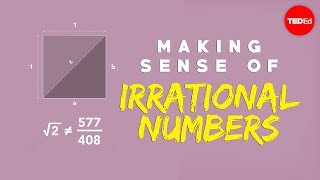◌◌◌ Hippasus
According to Pythagoras theorem the diagonal length of a square with each side measuring one unit would be square root of two. The assumption that square root of two could be expressed as a ratio of two integers deduces a contradiction.
http://qindex.info/i.php?x=6802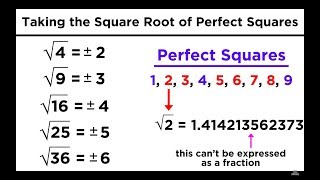07 Square Roots, Cube Roots, and Other Roots
Square Roots, Cube Roots, and Other Roots
http://qindex.info/i.php?x=499201 Hippasus
○ According to Pythagoras theorem the diagonal length of a square with each side measuring one unit would be square root of two. ○ The assumption that square root of two could be expressed as a ratio of two integers deduces a contradiction.
http://qindex.info/i.php?x=6563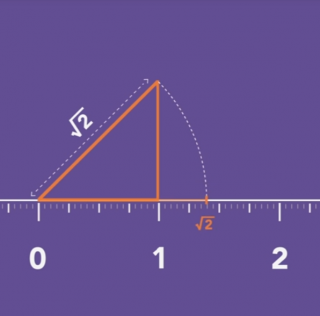√2 ≈ 1.414
According to Pythagoras theorem the diagonal length of a square with each side measuring one unit would be square root of two. The assumption that square root of two could be expressed as a ratio of two integers deduces a contradiction. #math
http://qindex.info/i.php?x=9592
○○○ 1054 ⪢⪢
This new rotating power-sharing agreement will be the root cause for several generations' numerous internal wars that will exhaust and divide the Kievan Rus'. #will_would
http://qindex.info/i.php?x=12942
---